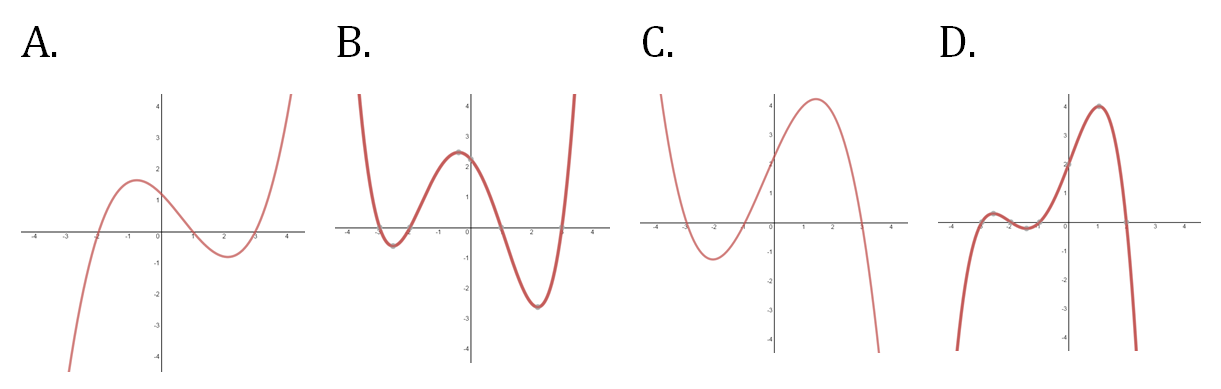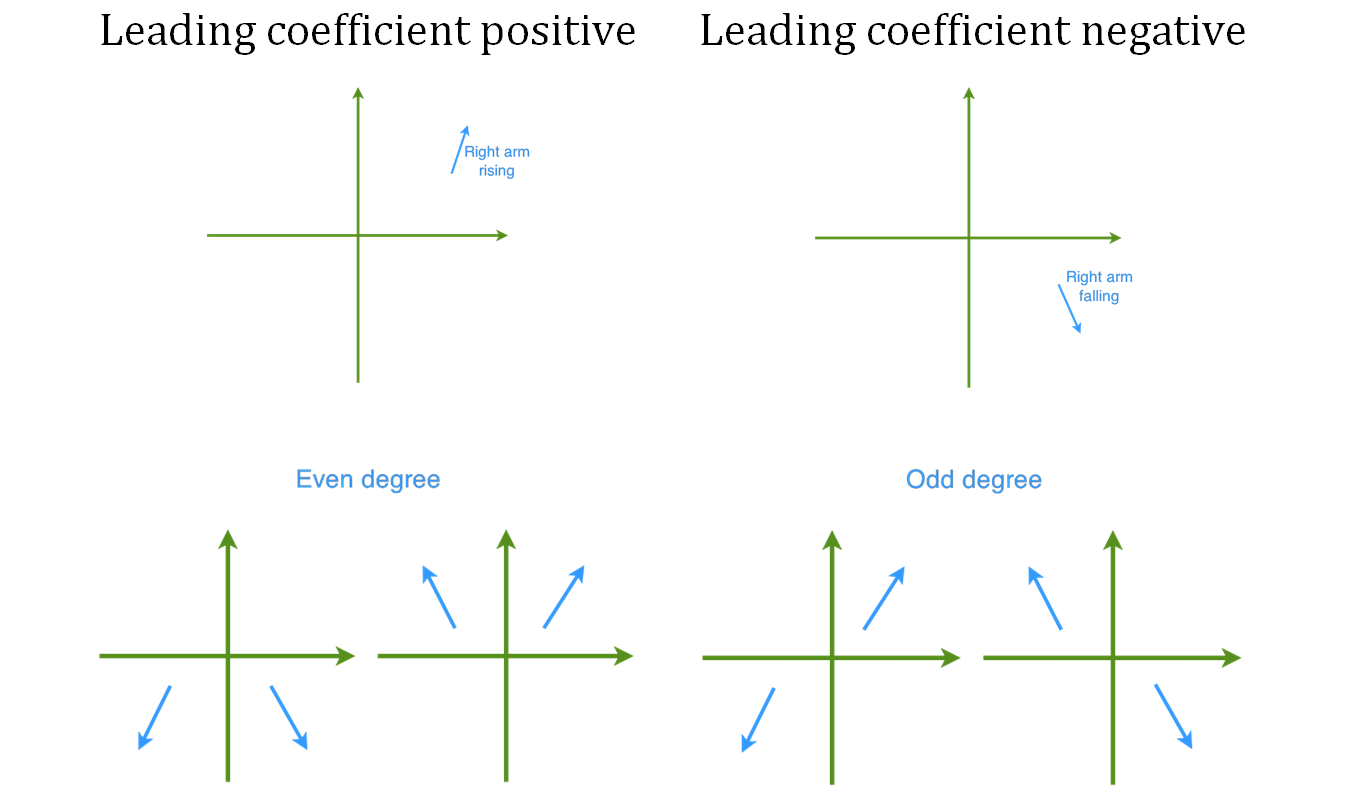# Characteristics of polynomial graphs

0/6

### Examples

#### Lessons

1. Investigating the Graphs of Polynomial Functions
Recognize the characteristics of polynomial graphs from the following quadratic and cubic functions:
i)
$P\left( x \right) = {x^2} - 3x - 10$
ii)
$P\left( x \right) = - {x^2} + 3x + 10$
iii)
$P\left( x \right) = {x^3} - 3{x^2} - 10x$
iv)
$P\left( x \right) = - {x^3} + 3{x^2} + 10x$
1. Graphing Polynomials
Sketch the following polynomials:
1. $P(x)=(x-3)(x-1)(x+2)(x+5)$
2. $P(x)=-(x-5)(x-2)(x+1)(x+4)(x+6)$
3. $P(x)=-x^2-2x+8$
4. $P(x)=x^3-8x^2+15x$
2. Identifying Polynomial Graphs
Which of the following could be the graph of a quadratic function with a negative leading coefficient?## Become a Member to Get More!

• #### Easily See Your ProgressWe track the progress you've made on a topic so you know what you've done. From the course view you can easily see what topics have what and the progress you've made on them. Fill the rings to completely master that section or mouse over the icon to see more details.

• #### Make Use of Our Learning Aids###### Practice Accuracy

See how well your practice sessions are going over time.

Stay on track with our daily recommendations.

• #### Earn Achievements as You LearnMake the most of your time as you use StudyPug to help you achieve your goals. Earn fun little badges the more you watch, practice, and use our service.

• #### Create and Customize Your AvatarPlay with our fun little avatar builder to create and customize your own avatar on StudyPug. Choose your face, eye colour, hair colour and style, and background. Unlock more options the more you use StudyPug.

###### Topic Basics